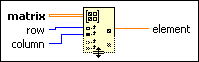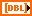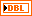# Get Matrix Elements Function

## LabVIEW 2018 Help

Edition Date: March 2018
Part Number: 371361R-01
View Product Info

### DOWNLOAD (Windows Only)LabVIEW 2016 HelpLabVIEW 2017 HelpLabVIEW 2018 HelpLabVIEW 2019 HelpLabVIEW 2020 Help

Owning Palette: Matrix Functions

Requires: Base Development System

Returns the elements of matrix at row and column.

The row and column inputs specify row and column indexes. If you wire scalar data to row and column, for example i for row and j for column, the function returns a scalar that contains the element at location (i, j).

The connector pane displays the default data types for this polymorphic function.matrix must be a 2D array of any numeric type or a real or complex matrix.row must be an integer, a floating-point numeric, a 1D array of integers, or a 1D array of a floating-point numeric. The default is a 1D array of integers that contains all row indexes.column must be an integer, a floating-point numeric, a 1D array of integers, or a 1D array of a floating-point numeric. The default is 0.element is the scalar, matrix, or 2D array that contains the matrix elements. The data type of element is the same as the data type of matrix.

## Get Matrix Elements Details

Add additional row and column inputs and element outputs by resizing the node.

If matrix is an empty matrix or array, element returns an invalid operation value at each exterior location.

If you do not wire row and column, element returns a column vector with the first column of matrix.

If you do not wire column and you wire a numeric scalar to row, element returns a row vector with the index of row. If you do not wire row and you wire a numeric scalar to column, element returns a column vector with the index of column.

If you wire a 1D array of indexes to either row or column and no value to the other index input, element returns a matrix.

If you wire a 1D array of numeric data to row or column, the function returns one matrix element for every location specified. These locations are determined by pairing each row index in row with each column index in column. For example, passing the array of integers {1, 2, 4} to row and the integer {5} to column retrieves matrix elements at indexes (1, 5), (2, 5), and (4, 5). In this case, the output is a row vector, or a 3 x 1 matrix, because the number of scalar elements wired to row is 3 and the number of scalars elements wired to column is 1.

### Wiring Empty Input Values

If you wire an empty 1D array to row or column, element returns an empty matrix or array with 0 in the corresponding row or column dimension.

### Indexing Outside Input Values

If you wire a negative value to row or column, the function returns an invalid operation value at each exterior location.

If you wire a value to row or column that is greater than the row or column dimension of matrix, the function returns an invalid operation value at each exterior location.

Not Helpful